# 8.1 - One Sample Proportion

8.1 - One Sample Proportion

One sample proportion tests and confidence intervals are covered in Section 6.1 of the Lock5 textbook.

In the last lesson you were introduced to the general concept of the Central Limit Theorem. The Central Limit Theorem states that if the sample size is sufficiently large then the sampling distribution will be approximately normally distributed for many frequently tested statistics, such as those that we have been working with in this course. When discussion proportions, we sometimes refer to this as the Rule of Sample Proportions. According to the Rule of Sample Proportions, if $$np\geq 10$$ and $$n(1-p) \geq 10$$ then the sampling distributing will be approximately normal. When constructing a confidence interval $$p$$ is not known but may be approximated using $$\widehat p$$. When conducting a hypothesis test, we check this assumption using the hypothesized proportion (i.e., the proportion in the null hypothesis).

If assumptions are met, the sampling distribution will have a standard error equal to $$\sqrt{\frac{p(1-p)}{n}}$$.

This method of constructing a sampling distribution is known as the normal approximation method.

If the assumptions for the normal approximation method are not met (i.e., if $$np$$ or $$n(1-p)$$ is not at least 10), then the sampling distribution may be approximated using a binomial distribution. This is known as the exact method. This course does not cover the exact method in detail, but you will see how these tests may be performed using Minitab.

# 8.1.1 - Confidence Intervals

8.1.1 - Confidence Intervals

On the following pages you will see how a confidence interval for a population proportion can be constructed by hand using the normal approximation method. Using Minitab, you will learn how to construct a confidence interval for a proportion using the normal approximation method or the exact method. When given the option, it is recommended that you use Minitab as opposed to performing calculations by hand.

# 8.1.1.1 - Normal Approximation Formulas

8.1.1.1 - Normal Approximation Formulas

For the following procedures, the assumption is that both $$np \geq 10$$ and $$n(1-p) \geq 10$$. When we're constructing confidence intervals $$p$$ is typically unknown, in which case we use $$\widehat{p}$$ as an estimate of $$p$$.

Note that $$n \widehat p$$ is the number of successes in the sample and $$n(1- \widehat p)$$ is the number of failures in the sample.

This means that our sample needs to have at least 10 "successes" and at least 10 "failures" in order to construct a confidence interval using the normal approximation method.

Below is the general form of a confidence interval.

General Form of Confidence Interval
$$sample\ statistic\pm\underbrace{(multiplier)\ (standard\ error)}_{\textbf{margin of error}}$$

The sample statistic here is the sample proportion, $$\widehat p$$. When using the normal approximation method the multiplier is taken from the standard normal distribution (i.e., z distribution).  And, the standard error is computed using $$\widehat p$$ as an estimate of $$p$$: $$\sqrt{\frac{\hat{p} (1-\hat{p})}{n}}$$. This leaves us with the following formula to construct a confidence interval for a population proportion:

Confidence Interval of $$p$$: Normal Approximation Method
$$\underbrace{\widehat{p}}_{\text{sample statistic}} \pm \overbrace{z^{*}}^{\text{multiplier}} \underbrace{\left (\sqrt{\frac{\hat{p}(1-\hat{p})}{n}}\right)}_{\text{standard error}}$$

## Finding the z* Multiplier

The value of the $$z^*$$ multiplier depends on the level of confidence. The multiplier for the confidence interval for a population proportion can be found using the standard normal distribution [i.e., z distribution, N(0,1)]. The most commonly used level of confidence is 95%. As shown on the probability distribution plot below, the multiplier associated with a 95% confidence interval is 1.960, often rounded to 2 (recall the Empirical Rule and 95% Rule).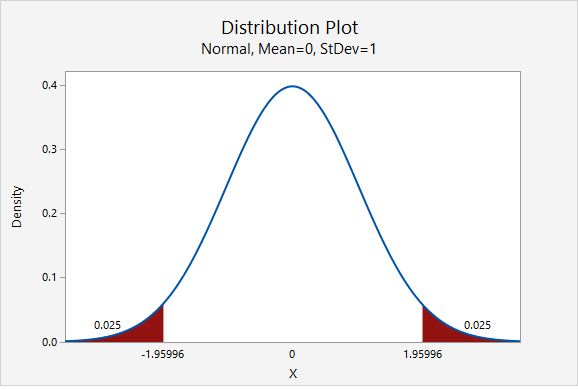Below is a table of frequently used $$z^*$$ multipliers.

Confidence level and corresponding multiplier.
Confidence Level $$z^*$$ Multiplier
90% 1.645
95% 1.960, often rounded to 2
98% 2.327
99% 2.576

The value of the multiplier increases as the confidence level increases. This leads to wider intervals for higher confidence levels. We are more confident of catching the population value when we use a wider interval.

# 8.1.1.1.1 - Video Example: PA Residency

8.1.1.1.1 - Video Example: PA Residency

# 8.1.1.1.2 - Video Example: Dog Ownership

8.1.1.1.2 - Video Example: Dog Ownership

In Spring 2016, a sample of 522 World Campus students were surveyed and asked if they own a dog. Of the 522 students in the sample, 273 said that they did have a dog. Construct a 95% confidence interval for the proportion of all World Campus students who have a dog.

# 8.1.1.1.3 - Video Example: Books

8.1.1.1.3 - Video Example: Books

# 8.1.1.1.4 - Example: Retirement

8.1.1.1.4 - Example: Retirement

In a representative sample of 1168 American adults, 747 said they were not financially prepared for retirement. Let's construct a 95% confidence interval to estimate the proportion of all American adults who are not financially prepared for retirement.

First, we need to check our assumptions that both $$n\widehat p \geq 10$$ and $$n(1-\widehat p) \geq 10$$.

$$\widehat{p}=\frac{747}{1168}=0.640$$

$$np=1168 (0.640) = 747$$ and $$n(1-p)=1168(1-0.640)=421$$

Both are greater than 10, so this assumption has been met. This means we can use the normal approximation method to construct this confidence interval.

Next, we can compute the standard error.

$$SE=\sqrt{\frac{\hat{p} (1-\hat{p})}{n}}=\sqrt{\frac{0.640 (1-0.640)}{1168}}=0.014$$

The $$z^*$$ multiplier for a 95% confidence interval is 1.960

The formula for a confidence interval for a proportion is $$\widehat{p}\pm z^* (SE)$$

$$0.640\pm 1.960(0.014)=0.640\pm0.028=[0.612, \;0.668]$$

We are 95% confident that between 61.2% and 66.8% of all American adults are not financially prepared for retirement.

What if we wanted a 99% confidence interval?

Let’s think about how our interval will change. The 99% confidence interval will be wider than the 95% confidence interval. In order to increase our level of confidence, we will need to expand the interval.

In terms of computing the 99% confidence interval, we will use the same point estimate $$\widehat{p}$$ and the same standard error. Only the multiplier will change. From the plot below, we see that the $$z^*$$ multiplier for a 99% confidence interval is 2.576.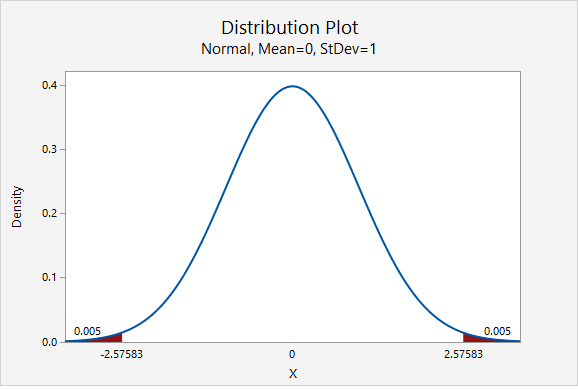$$99\%\;C.I.:\;0.640\pm 2.576 (0.014)=0.0640\pm 0.036=[0.604, \; 0.676]$$

We are 99% confidence that between 60.4% and 67.6% of all American adults are not financially prepared for retirement.

# 8.1.1.2 - Minitab: Confidence Interval for a Proportion

8.1.1.2 - Minitab: Confidence Interval for a Proportion

Before we can construct a confidence interval for a proportion we must first determine if we should use the exact method or the normal approximation method. Recall that if $$np \geq 10$$ and $$n(1-p) \geq 10$$ then the sampling distribution can be approximated by a normal distribution. Since we don't have the population proportion ($$p$$), we using $$\widehat p$$ as an estimate. Note that $$n\widehat p$$ is the number of successes in the sample and $$n(1-\widehat p)$$ is the number of failures in the sample.

If this assumption has not been met, then the sampling distribution is constructed using a binomial distribution which Minitab refers to as the "exact method."

To check this assumption we can construct a frequency table. You first learned how to construct a frequency table in Lesson 2.1.1.2.1 of these online notes. Here is another example:

## Minitab® – Frequency Tables

To create a frequency table of dog ownership in Minitab:

1. Open the data set:
2. From the toolbar in Minitab, select Stat > Tables > Tally Individual Variables
3. Double click the variable Dog in the box on the left to insert the variable into the Variable box
4. Under Display, choose Counts
5. Click OK

This should result in the following frequency table:

Tally
Dog Count
No 252
Yes 272
N= 524
*= 1

From the frequency table above we can see that there were at least 10 "successes" and at least 10 "failures" in the sample. In this example a success is defined as answering "yes" to the question "do you own a dog?" A failure is defined as answering "no." Because both $$n \widehat p \geq 10$$ and $$n(1- \widehat p) \geq 10$$, the normal approximation method may be used. In Minitab, the exact method is the default method. If there are at least 10 successes and at least 10 failures, then you need to change the method to the normal approximation method.

## Minitab® – Confidence Interval for a Proportion (Normal Approximation)

To create a 95% confidence interval of dog ownership using the normal approximation method in Minitab:

1. Open the data set: fall2016stdata.mpx
2. In Minitab, select Stat > Basic Statistics > 1-Proportion
3. In this case we have our data in the Minitab worksheet so we will use the default One or more samples each in a column.
4. Double click the variable Dog in the box on the left to insert the variable into the box.
5. Select Options
6. The default Confidence level is 95
7. Change the Method to Normal approximation because the assumption of $$n \widehat p \geq 10$$ and $$n(1- \widehat p) \geq 10$$ has been met
8. Click OK

This should result in the following output:

#### Method

Event: Dog = Yes

p: proportion where Dog = Yes

Normal approximation is used for this analysis.

Descriptive Statistics
N Event Sample p 95% CI for p
524 272 0.519084 (0.476304, 0.561863)

## What if the assumption is not met?

If the number of successes or the number of failures in the sample is less than 10, then the exact method should be used instead of the normal approximation method. In Minitab, this means that in step 8 above the default setting of Exact method should not be changed.

What if we have summarized data and not data in a Minitab worksheet?

If you do not have a Minitab worksheet filled with data concerning individuals, but instead have summarized data (e.g., the number of successes and the number of failures), you would not load the data set, but in step 3 you would select Summarized data. For Number of events, enter the number of successes (i.e., $$n \widehat p$$) and for Number of trials enter the total sample size (i.e., $$n$$).

# 8.1.1.2.1 - Example with Summarized Data

8.1.1.2.1 - Example with Summarized Data

## Example: Lactose Intolerance

In a sample of 100 African American adults, 70 were identified as having some level of lactose intolerance. Compute a 95% confidence interval to estimate the proportion of all African American adults who have some level of lactose intolerance.

To create a 95% confidence interval of dog ownership using the normal approximation method in Minitab:

In Minitab, select Stat > Basic Statistics > 1-Proportion
1. In this case we have summarized data so select Summarized data in the dropdown.
2. For number of events, add 70 and for number of trials add 100.
3. Select Options
4. The default Confidence level is 95.
5. Change the Method to Normal approximation because the assumption of $$n \widehat p \geq 10$$ and $$n(1- \widehat p) \geq 10$$ has been met
6. Click OK and OK.

This should result in the following output:

#### Method

p: event proportion

Normal approximation is used for this analysis.

Descriptive Statistics
N Event Sample p 95% CI for p
100 70 0.700000 (0.610183, 0.789817)

# 8.1.1.2.2 - Example with Summarized Data

8.1.1.2.2 - Example with Summarized Data

## Example: Dieting

At the beginning of the Fall 2016 semester a representative sample of World Campus STAT 200 students was surveyed. The students were asked if they were currently dieting to lose weight. In the sample of 524 students, 184 said that they were dieting to lose weight. Construct a 95% confidence interval for the proportion of all World Campus STAT 200 students who are dieting to lose weight.

In Minitab, select Stat > Basic Statistics > 1-Proportion
1. In this case we have summarized data so select Summarized data in the dropdown.
2. For number of events, add 184 and for number of trials add 524.
3. Select Options
4. The default Confidence level is 95.
5. Change the Method to Normal approximation because the assumption of $$n \widehat p \geq 10$$ and $$n(1- \widehat p) \geq 10$$ has been met
6. Click OK and OK.

This should result in the following output:

#### Method

p: event proportion

Normal approximation is used for this analysis.

Descriptive Statistics
N Event Sample p 95% CI for p
524 184 0.351145 (0.310276, 0.392015)

# 8.1.1.3 - Computing Necessary Sample Size

8.1.1.3 - Computing Necessary Sample Size

When we begin a study to estimate a population parameter we typically have an idea as how confident we want to be in our results and within what degree of accuracy. This means we get started with a set level of confidence and margin of error. We can use these pieces to determine a minimum sample size needed to produce these results by using algebra to solve for $$n$$:

Finding Sample Size for Estimating a Population Proportion
$$n=\left ( \frac{z^*}{M} \right )^2 \tilde{p}(1-\tilde{p})$$

$$M$$ is the margin of error
$$\tilde p$$ is an estimated value of the proportion

If we have no preconceived idea of the value of the population proportion, then we use $$\tilde{p}=0.50$$ because it is most conservative and it will give use the largest sample size calculation.

## Example: No Estimate

We want to construct a 95% confidence interval for $$p$$ with a margin of error equal to 4%.

Because there is no estimate of the proportion given, we use $$\tilde{p}=0.50$$ for a conservative estimate.

For a 95% confidence interval, $$z^*=1.960$$

$$n=\left ( \frac{1.960}{0.04} \right )^2 (0.5)(1-0.5)=600.25$$

This is the minimum sample size, therefore we should round up to 601. In order to construct a 95% confidence interval with a margin of error of 4%, we should obtain a sample of at least $$n=601$$.

## Example: Estimate Known

We want to construct a 95% confidence interval for $$p$$ with a margin of error equal to 4%. What if we knew that the population proportion was around 0.25?

The $$z^*$$ multiplier for a 95% confidence interval is 1.960. Now, we have an estimate to include in the formula:

$$n=\left ( \frac{1.960}{0.04} \right )^2 (0.25)(1-0.25)=450.188$$

Again, we should round up to 451. In order to construct a 95% confidence interval with a margin of error of 4%, given $$\tilde{p}=.25$$, we should obtain a sample of at least $$n=451$$.

Note that when we changed $$\tilde{p}$$ in the formula from .50 to .25, the necessary sample size decreased from $$n=601$$ to $$n=451$$.

# 8.1.2 - Hypothesis Testing

8.1.2 - Hypothesis Testing

A hypothesis test for a proportion is used when you are comparing one group to a known or hypothesized population proportion value. In other words, you have one sample with one categorical variable. The hypothesized value of the population proportion is symbolized by $$p_0$$ because this is the value in the null hypothesis ($$H_0$$).

If $$np_0 \ge 10$$ and $$n(1-p_0) \ge 10$$ then the distribution of sample proportions is approximately normal and can be estimated using the normal distribution. That sampling distribution will have a mean of $$p_0$$ and a standard deviation (i.e., standard error) of $$\sqrt{\frac{p_0 (1-p_0)}{n}}$$

Recall that the standard normal distribution is also known as the z distribution. Thus, this is known as a "single sample proportion z test" or "one sample proportion z test."

If $$np_0 < 10$$ or $$n(1-p_0) < 10$$ then the distribution of sample proportions follows a binomial distribution. We will not be conducting this test by hand in this course, however you will learn how this can be conducted using Minitab using the exact method.

# 8.1.2.1 - Normal Approximation Method Formulas

8.1.2.1 - Normal Approximation Method Formulas

Here we will be using the five step hypothesis testing procedure to compare the proportion in one random sample to a specified population proportion using the normal approximation method.

1. Check assumptions and write hypotheses

In order to use the normal approximation method, the assumption is that both $$n p_0 \geq 10$$ and $$n (1-p_0) \geq 10$$. Recall that $$p_0$$ is the population proportion in the null hypothesis.

Research Question Is the proportion different from $$p_0$$? Is the proportion greater than $$p_0$$? Is the proportion less than $$p_0$$?
Null Hypothesis, $$H_{0}$$ $$p=p_0$$ $$p= p_0$$ $$p= p_0$$
Alternative Hypothesis, $$H_{a}$$ $$p\neq p_0$$ $$p> p_0$$ $$p< p_0$$
Type of Hypothesis Test Two-tailed, non-directional Right-tailed, directional Left-tailed, directional

Where $$p_0$$ is the hypothesized population proportion that you are comparing your sample to.

2. Calculate the test statistic

When using the normal approximation method we will be using a z test statistic. The z test statistic tells us how far our sample proportion is from the hypothesized population proportion in standard error units. Note that this formula follows the basic structure of a test statistic that you learned last week: $$test\;statistic=\frac{sample\;statistic-null\;parameter}{standard\;error}$$

Test statistic: One Group Proportion
$$z=\dfrac{\widehat{p}- p_0 }{\sqrt{\frac{p_0 (1- p_0)}{n}}}$$

$$\widehat{p}$$ = sample proportion
$$p_{0}$$ = hypothesize population proportion
$$n$$ = sample size

3. Determine the p-value

Given that the null hypothesis is true, the p value is the probability that a randomly selected sample of n would have a sample proportion as different, or more different, than the one in our sample, in the direction of the alternative hypothesis. We can find the p value by mapping the test statistic from step 2 onto the z distribution.

Note that p-values are also symbolized by $$p$$. Do not confuse this with the population proportion which shares the same symbol.

We can look up the $$p$$-value using Minitab by constructing the sampling distribution.  Because we are using the normal approximation here, we have a $$z$$ test statistic that we can map onto the $$z$$ distribution. Recall, the z distribution is a normal distribution with a mean of 0 and standard deviation of 1. If we are conducting a one-tailed (i.e., right- or left-tailed) test, we look up the area of the sampling distribution that is beyond our test statistic. If we are conducting a two-tailed (i.e., non-directional) test there is one additional step: we need to multiple the area by two to take into account the possibility of being in the right or left tail.

4. Make a decision

We can decide between the null and alternative hypotheses by examining our p-value. If $$p \leq \alpha$$ reject the null hypothesis. If $$p>\alpha$$ fail to reject the null hypothesis. Unless stated otherwise, assume that $$\alpha=.05$$.

When we reject the null hypothesis our results are said to be statistically significant.

5. State a "real world" conclusion

Based on our decision in step 4, we will write a sentence or two concerning our decision in relation to the original research question.

# 8.1.2.1.1 - Video Example: Male Babies

8.1.2.1.1 - Video Example: Male Babies

# 8.1.2.1.2 - Example: Handedness

8.1.2.1.2 - Example: Handedness

Research Question: Are more than 80% of American's right handed?

In a sample of 100 Americans, 87 were right handed.

1. Check assumptions and write hypotheses

$$np_0 = 100(0.80)=80$$

$$n(1-p_0) = 100 (1-0.80) = 20$$

Both $$np_0$$ and $$n(1-p_0)$$ are at least 10 so we can use the normal approximation method.

This is a right-tailed test because we want to know if the proportion is greater than 0.80.

$$H_{0}\colon p=0.80$$
$$H_{a}\colon p>0.80$$

2. Calculate the test statistic
Test statistic: One Group Proportion

$$z=\dfrac{\widehat{p}- p_0 }{\sqrt{\frac{p_0 (1- p_0)}{n}}}$$

$$\widehat{p}$$ = sample proportion
$$p_{0}$$ = hypothesize population proportion
$$n$$ = sample size

$$\widehat{p}=\dfrac{87}{100}=0.87$$, $$p_{0}=0.80$$, $$n=100$$

$$z= \dfrac{\widehat{p}- p_0 }{\sqrt{\frac{p_0 (1- p_0)}{n}}}= \dfrac{0.87-0.80}{\sqrt{\frac{0.80 (1-0.80)}{100}}}=1.75$$

Our $$z$$ test statistic is 1.75.

3. Determine the p-value associated with the test statistic

This is a right-tailed test so we need to find the area to the right of the test statistic, $$z=1.75$$, on the z distribution.

Using Minitab , we find the probability $$P(z\geq1.75)=0.0400592$$ which may be rounded to $$p\; value=0.0401$$.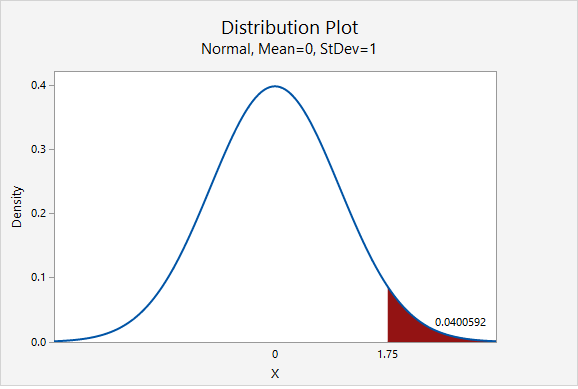4. Make a decision

$$p\leq .05$$, therefore our decision is to reject the null hypothesis

5. State a "real world" conclusion

Yes, there is statistical evidence to state that more than 80% of all Americans are right handed.

# 8.1.2.1.3 - Example: Ice Cream

8.1.2.1.3 - Example: Ice Cream

Research Question: Is the percentage of Creamery customers who prefer chocolate ice cream over vanilla less than 80%?

In a sample of 50 customers 60% preferred chocolate over vanilla.

1. Check assumptions and write hypotheses

$$np_0 = 50(0.80) = 40$$

$$n(1-p_0)=50(1-0.80) = 10$$

Both $$np_0$$ and $$n(1-p_0)$$ are at least 10. We can use the normal approximation method.

This is a left-tailed test because we want to know if the proportion is less than 0.80.

$$H_{0}\colon p=0.80$$
$$H_{a}\colon p<0.80$$

2. Calculate the test statistic
Test statistic: One Group Proportion

$$z=\dfrac{\widehat{p}- p_0 }{\sqrt{\frac{p_0 (1- p_0)}{n}}}$$

$$\widehat{p}$$ = sample proportion
$$p_{0}$$ = hypothesize population proportion
$$n$$ = sample size

$$\widehat{p}=0.60$$, $$p_{0}=0.80$$, $$n=50$$

$$z= \dfrac{\widehat{p}- p_0 }{\sqrt{\frac{p_0 (1- p_0)}{n}}}= \dfrac{0.60-0.80}{\sqrt{\frac{0.80 (1-0.80)}{50}}}=-3.536$$

Our $$z$$ test statistic is -3.536.

3. Determine the p-value

This is a left-tailed test so we need to find the area to the right of our test statistic, $$z=-3.536$$.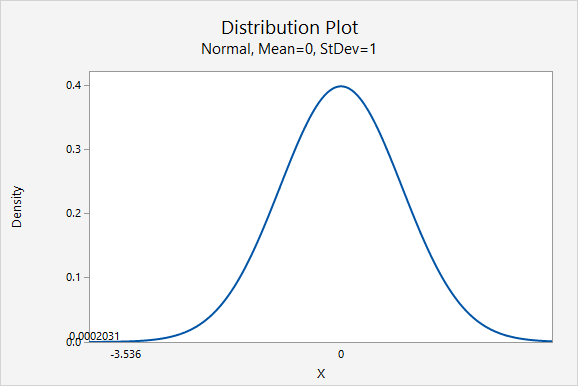From the Minitab output above, the p-value is 0.0002031

4. Make a decision

$$p \leq.05$$, therefore our decision is to reject the null hypothesis.

5. State a "real world" conclusion

Yes, there is evidence that the percentage of all Creamery customers who prefer chocolate ice cream over vanilla is less than 80%.

# 8.1.2.1.4 - Example: Overweight Citizens

8.1.2.1.4 - Example: Overweight Citizens

According to the Center for Disease Control (CDC), the percent of adults 20 years of age and over in the United States who are overweight is 69.0% (see http://www.cdc.gov/nchs/fastats/obesity-overweight.htm). One city’s council wants to know if the proportion of overweight citizens in their city is different from this known national proportion. They take a random sample of 150 adults 20 years of age or older in their city and find that 98 are classified as overweight. Let’s use the five step hypothesis testing procedure to determine if there is evidence that the proportion in this city is different from the known national proportion.

1. Check assumptions and write hypotheses

$$np_0 =150 (0.690)=103.5$$

$$n (1-p_0) =150 (1-0.690)=46.5$$

Both $$n p_0$$ and $$n (1-p_0)$$ are at least 10, this assumption has been met.

Research question: Is this city’s proportion of overweight individuals different from 0.690?

This is a non-directional test because our question states that we are looking for a differences as opposed to a specific direction. This will be a two-tailed test.

$$H_{0}\colon p=0.690$$
$$H_{a}\colon p\neq 0.690$$

2. Calculate the test statistic
Test statistic: One Group Proportion

$$z=\dfrac{\widehat{p}- p_0 }{\sqrt{\frac{p_0 (1- p_0)}{n}}}$$

$$\widehat{p}$$ = sample proportion
$$p_{0}$$ = hypothesize population proportion
$$n$$ = sample size

$$\widehat{p}=\dfrac{98}{150}=.653$$

$$z =\dfrac{0.653- 0.690 }{\sqrt{\frac{0.690 (1- 0.690)}{150}}} = -0.980$$

Our test statistic is $$z=-0.980$$

3. Determine the p-value

This is a non-directional (i.e., two-tailed) test, so we need to find the area under the z distribution that is more extreme than $$z=-0.980$$.

In Minitab, we find the proportion of a normal curve beyond $$\pm0.980$$: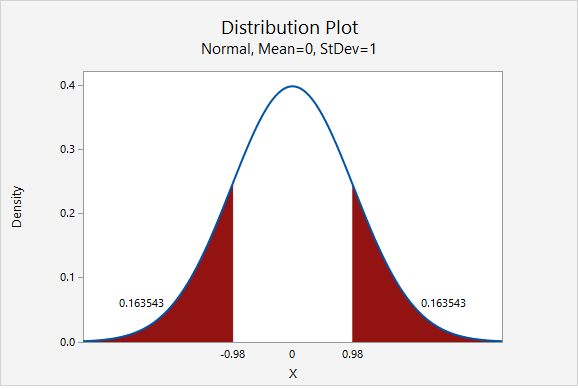$$p-value=0.163543+0.163543=0.327086$$

4. Make a decision

$$p>\alpha$$, therefore we fail to reject the null hypothesis

5. State a "real world" conclusion

There is not sufficient evidence to state that the proportion of citizens of this city who are overweight is different from the national proportion of 0.690.

# 8.1.2.2 - Minitab: Hypothesis Tests for One Proportion

8.1.2.2 - Minitab: Hypothesis Tests for One Proportion

A hypothesis test for one proportion can be conducted in Minitab. This can be done using raw data or summarized data.

• If you have a data file with every individual's observation, then you have raw data.
• If you do not have each individual observation, but rather have the sample size and number of successes in the sample, then you have summarized data.

The next two pages will show you how to use Minitab to conduct this analysis using either raw data or summarized data.

Note that the default method for constructing the sampling distribution in Minitab is to use the exact method.  If $$np_0 \geq 10$$ and $$n(1-p_0) \geq 10$$ then you will need to change this to the normal approximation method.  This must be done manually. Minitab will use the method that you select, it will not check assumptions for you!

# 8.1.2.2.1 - Minitab: 1 Proportion z Test, Raw Data

8.1.2.2.1 - Minitab: 1 Proportion z Test, Raw Data

If you have data in a Minitab worksheet, then you have what we call "raw data."  This is in contrast to "summarized data" which you'll see on the next page.

In order to use the normal approximation method both $$np_0 \geq 10$$ and $$n(1-p_0) \geq 10$$. Before we can conduct our hypothesis test we must check this assumption to determine if the normal approximation method or exact method should be used. This must be checked manually. Minitab will not check assumptions for you.

In the example below, we want to know if there is evidence that the proportion of students who are male is different from 0.50.

$$n=226$$ and $$p_0=0.50$$

$$np_0 = 226(0.50)=113$$ and $$n(1-p_0) = 226(1-0.50)=113$$

Both $$np_0 \geq 10$$ and $$n(1-p_0) \geq 10$$ so we can use the normal approximation method.

## Minitab® – Conducting a One Sample Proportion z Test: Raw Data

Research question: Is the proportion of students who are male different from 0.50?

1. Open Minitab file:
2. In Minitab, select Stat > Basic Statistics > 1 Proportion
3. Select One or more samples, each in a column from the dropdown
4. Double-click the variable Biological Sex to insert it into the box
5. Check the box next to Perform hypothesis test and enter 0.50 in the Hypothesized proportion box
6. Select Options
7. Use the default Alternative hypothesis setting of Proportion ≠ hypothesized proportion value
8. Use the default Confidence level of 95
9. Select Normal approximation method
10. Click OK and OK

The result should be the following output:

#### Method

Event: Biological Sex = Male
p: proportion where Biological Sex = Male
Normal approximation is used for this analysis.

Descriptive Statistics
N Event Sample p 95% CI for p
226 99 0.438053 (0.373368, 0.502738)
Null hypothesis H 0: p = 0.5 H 1: p ≠ 0.5
Z-Value P-Value
-1.86 0.063

## Summary of Results

We could summarize these results using the five-step hypothesis testing procedure:

1. Check assumptions and write hypotheses

$$np_0 = 226(0.50)=113$$ and $$n(1-p_0) = 226(1-0.50)=113$$ therefore the normal approximation method will be used.

$$H_0\colon p = 0.50$$

$$H_a\colon p \ne 0.50$$

2. Calculate the test statistic

From the Minitab output, $$z$$ = -1.86

3. Determine the p-value

From the Minitab output, $$p$$ = 0.0625

4. Make a decision

$$p > \alpha$$, fail to reject the null hypothesis

5. State a "real world" conclusion

There is NOT enough evidence that the proportion of all students in the population who are male is different from 0.50.

# 8.1.2.2.2 - Minitab: 1 Sample Proportion z test, Summary Data

8.1.2.2.2 - Minitab: 1 Sample Proportion z test, Summary Data

## Example: Overweight

The following example uses a scenario in which we want to know if the proportion of college women who think they are overweight is less than 40%. We collect data from a random sample of 129 college women and 37 said that they think they are overweight.

First, we should check assumptions to determine if the normal approximation method or exact method should be used:

$$np_0=129(0.40)=51.6$$ and $$n(1-p_0)=129(1-0.40)=77.4$$ both values are at least 10 so we can use the normal approximation method.

## Minitab® – Performing a One Proportion z Test with Summarized Data

To perform a one sample proportion z test with summarized data in Minitab:

1. In Minitab, select Stat > Basic Statistics > 1 Proportion
2. Select Summarized data from the dropdown
3. For number of events, add 37 and for number of trials add 129.
4. Check the box next to Perform hypothesis test and enter 0.40 in the Hypothesized proportion box
5. Select Options
6. Use the default Alternative hypothesis setting of Proportion < hypothesized proportion value
7. Use the default Confidence level of 95
8. Select Normal approximation method
9. Click OK and OK

The result should be the following output:

#### Method

Event: Event proportion
Normal approximation is used for this analysis.

Descriptive Statistics
N Event Sample p 95% Upper Bound for p
129 37 0.286822 0.352321
Null hypothesis H 0: p = 0.4 H 1: p < 0.4
Z-Value P-Value
-2.62 0.004

## Summary of Results

We could summarize these results using the five-step hypothesis testing procedure:

1. Check assumptions and write hypotheses

$$np_0=129(0.40)=51.6$$ and $$n(1-p_0)=129(1-0.40)=77.4$$ both values are at least 10 so we can use the normal approximation method.

$$H_0\colon p = 0.40$$

$$H_a\colon p < 0.40$$

2. Calculate the test statistic

From output, $$z$$ = -2.62

3. Determine the p-value

From output, $$p$$ = 0.004

4. Make a decision

$$p \leq \alpha$$, reject the null hypothesis

5. State a "real world" conclusion

There is evidence that the proportion of women in the population who think they are overweight is less than 40%.

# 8.1.2.2.2.1 - Minitab Example: Normal Approx. Method

8.1.2.2.2.1 - Minitab Example: Normal Approx. Method

## Example: Gym membership

Research question: Are less than 50% of all individuals with a membership at one gym female?

A simple random sample of 60 individuals with a membership at one gym was collected. Each individual's biological sex was recorded. There were 24 females.

First we have to check the assumptions:

np = 60 (0.50) = 30

n(1-p) = 60(1-0.50) = 30

The assumptions are met to use the normal approximation method.

To perform a one sample proportion z test with summarized data in Minitab:

1. In Minitab, select Stat > Basic Statistics > 1 Proportion
2. Select Summarized data from the dropdown
3. For number of events, add 24 and for number of trials add 60.
4. Check the box next to Perform hypothesis test and enter 0.50 in the Hypothesized proportion box
5. Select Options
6. Use the default Alternative hypothesis setting of Proportion < hypothesized proportion value
7. Use the default Confidence level of 95
8. Select Normal approximation method
9. Click OK and OK

The result should be the following output:

#### Method

Event: Event proportion
Normal approximation is used for this analysis.

Descriptive Statistics
N Event Sample p 95% Upper Bound for p
60 24 0.400000 0.504030
Null hypothesis H 0: p = 0.5 H 1: p < 0.5
Z-Value P-Value
-1.55 0.061

We could summarize these results using the five-step hypothesis testing procedure:

1. Check assumptions and write hypotheses

$$np_0=60(0.50)=30$$ and $$n(1-p_0)=60(1-0.50)=30$$ both values are at least 10 so we can use the normal approximation method.

$$H_0\colon p = 0.50$$

$$H_a\colon p < 0.50$$

2. Calculate the test statistic

From output, $$z$$ = -1.55

3. Determine the p-value

From output, $$p$$ = 0.061

4. Make a decision

$$p \geq \alpha$$, fail to reject the null hypothesis

5. State a "real world" conclusion

There is not enough evidence to support the alternative that the proportion of women memberships at this gym is less than 50%.

  Link ↥ Has Tooltip/Popover Toggleable Visibility